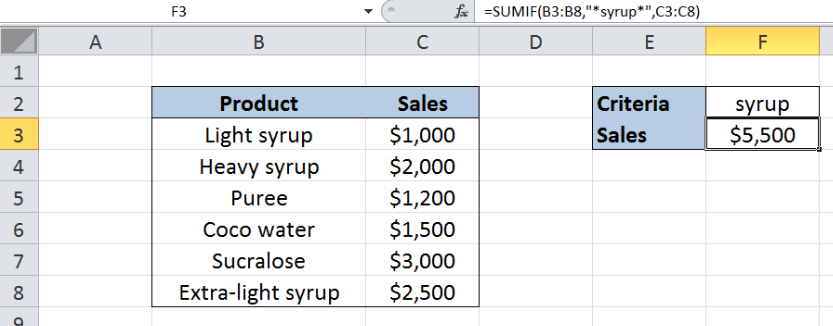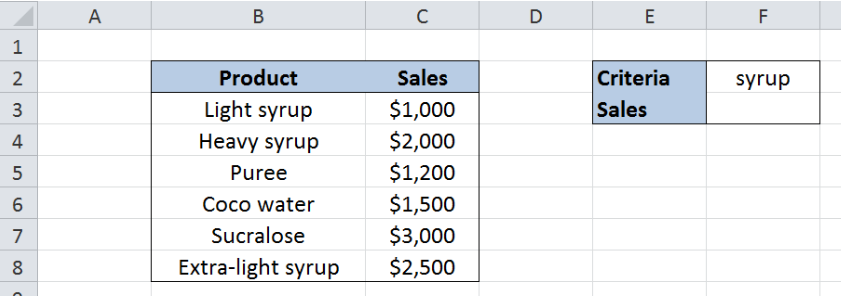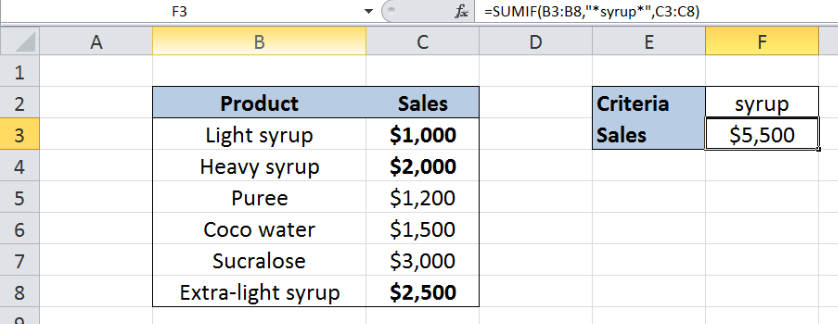Get instant live expert help with Excel or Google Sheets“My Excelchat expert helped me in less than 20 minutes, saving me what would have been 5 hours of work!”

#### Post your problem and you’ll get expert help in seconds.

Your message must be at least 40 characters
Our professional experts are available now. Your privacy is guaranteed.

# Using SUMIF to Add Cells If They Contain Certain Text

While working with Excel, we are able to sum values that satisfy a given criteria by using the SUMIF function. This step by step tutorial will assist all levels of Excel users in summing values that may contain certain text.Figure 1.  Final result: Using SUMIF to add cells containing certain text

Final formula: `=SUMIF(B3:B8,"*syrup*",C3:C8)`

## Syntax of the SUMIF Function

SUMIF sums the values in a specified range, based on one given criteria

`=SUMIF(range,criteria, [sum_range])`

The parameters are:

• Range: the data range that we will evaluate using the criteria
• Criteria: the criteria or condition that determines which cells will be added
• Sum_range: the cells that will be added; if left blank, “sum_range” = “range” which means that the range of data that will be added is the same range of data evaluated

## Setting up the Data

Our table has two columns: Product (column B) and Sales (column C).  Cell F2 contains our criteria “syrup”.  We want to obtain the total sales for products containing the text “syrup” in the product name.  We enter the result in cell F3.Figure 2.  Sample data to add cells containing the text “syrup”

## Sum of sales for “syrup” using SUMIF

We want to determine the total sales for products containing the text “syrup” using SUMIF.  Let us follow these steps:

Step 1. Select cell F3

Step 2. Enter the formula: `=``SUMIF(B3:B8,"*syrup*",C3:C8)`

Step 3. Press Enter

The range of the data we want to evaluate is B3:B8. We want to sum the sales in C3:C8 that satisfy the given criteria.

We want to add the sales of any product in column B containing the text “syrup”.  Our criteria “*syrup*includes all cells which may have texts before or after the word syrup.  The asterisk * is used as a wildcard in Excel, which means any number of characters.

The products that satisfy our criteria are “Light syrup”, Heavy syrup” and “Extra-light syrup”.  As a result, the total sales for products with “syrup” in cell F3 is \$ 5,500.Figure 3.  Entering the formula using SUMIF to add cells containing the text “syrup”

Most of the time, the problem you will need to solve will be more complex than a simple application of a formula or function. If you want to save hours of research and frustration, try our live Excelchat service! Our Excel Experts are available 24/7 to answer any Excel question you may have. We guarantee a connection within 30 seconds and a customized solution within 20 minutes.

Solution examplesamending a formula so that there is another criteria and if it does not meet this criteria then we stick with the original criteria
Solved by A. B. in 40 minsI need Column D to = Column C - Column A only if Column B has a 'Date' entered.
Solved by I. B. in 11 minsHello, I need help trying to create a sum of numerical values on a spreadsheet in which the only values that apply to the sum are when the status of another cell is a certain value (e.g. value is not in sum when status: closed, value is in sum when status: open)
Solved by T. W. in 18 minsI am looking for a formula to SUM column L, if Column A matched the relevant date and column C matches the name
Solved by Z. Y. in 16 minsI need a formula that is essentially saying IF this range of cells contain a certain name, add the number in another cell on the same row, and them sum that number in a different cell..!
Solved by O. H. in 44 mins